# python数据分析：分类分析（classification analysis）

## 分类分析使用场景：

#### 提炼应用规则：

• 对沉默会员做会员重新激活，应该挑选具有何种特征会员
• 商品选取何种促销活动清仓
• 那些广告更适合VIP商家的投放需求

## 分类分析算法的选取：

• 文本分类时用到最多的是朴素贝叶斯
• 训练集比较小，那么选择高偏差且低方差的分类算法效果逢高，如朴素贝叶斯、支持向量机、这些算法不容易过拟合。
• 训练集比较大，选取何种方法都不会显著影响准去度
• 省时好操作选着用支持向量机，不要使用神经网络
• 重视算法准确度，那么选择算法精度高的算法，例如支持向量机、随机森林。
• 想得到有关预测结果的概率信息，使用逻辑回归
• 需要清洗的决策规则，使用决策树

## python代码实现决策树算法

import numpy as np
import pandas as pd
from sklearn.model_selection import train_test_split
from sklearn.tree import DecisionTreeClassifier, export_graphviz
# 导入评估指标模块
from sklearn.metrics import accuracy_score, auc, confusion_matrix, f1_score, precision_score, recall_score, roc_curve
# 导入表格库
import prettytable
# 导入dot插件库
import pydotplus
import matplotlib.pyplot as plt
import seaborn as sns
%matplotlib inline

# 数据导入
df = pd.read_csv('https://raw.githubusercontent.com/ffzs/dataset/master/glass.csv', usecols=['Na','Ca','Type'])

v# 为了决策树图示简洁我们尽量减少分类，和特征值
dfs = df[df.Type < 3]

# 获取特征值
X = dfs[dfs.columns[:-1]].values
# 获取标签值
y = dfs['Type'].values - 1

# 将数据37分为测试集合训练集
X_train, X_test, y_train, y_test = train_test_split(X, y, test_size=0.3, random_state=2018)

#### 模型训练 ####
# 决策树模型
dt_model = DecisionTreeClassifier(random_state=2018)

# 训练模型
dt_model.fit(X_train, y_train)

# 对测试集做预测
pre_y = dt_model.predict(X_test)

####模型评估####
# 混淆矩阵
confusion_m = confusion_matrix(y_test, pre_y)

df_confusion_m = pd.DataFrame(confusion_m, columns=['0', '1'], index=['0', '1'])

df_confusion_m.index.name = 'Real'
df_confusion_m.columns.name = 'Predict'

df_confusion_m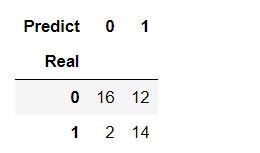# 获取决策树的预测概率
y_score = dt_model.predict_proba(X_test)

# ROC
fpr, tpr, thresholds = roc_curve(y_test, y_score[:, ])

# AUC
auc_s = auc(fpr, tpr)

# 准确率
accuracy_s = accuracy_score(y_test, pre_y)

# 精准度
precision_s = precision_score(y_test, pre_y)

# 召回率
recall_s = recall_score(y_test, pre_y)

# F1得分
f1_s = f1_score(y_test, pre_y)

# 评估数据制表
df_metrics = pd.DataFrame([[auc_s, accuracy_s, precision_s, recall_s, f1_s]], columns=['auc', 'accuracy', 'precision', 'recall', 'f1'], index=['结果'])

df_metrics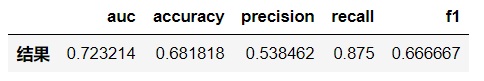#### 可视化ROC#####
plt.figure(figsize=(8, 7))
plt.plot(fpr, tpr, label='ROC')  # 画出ROC曲线
plt.plot([0, 1], [0, 1], linestyle='--', color='k', label='random chance')
# 画出随机状态下的准确率线
plt.title('ROC')  # 子网格标题
plt.xlabel('false positive rate')  # X轴标题
plt.ylabel('true positive rate')  # y轴标题
plt.legend(loc=0)
plt.savefig('x.png')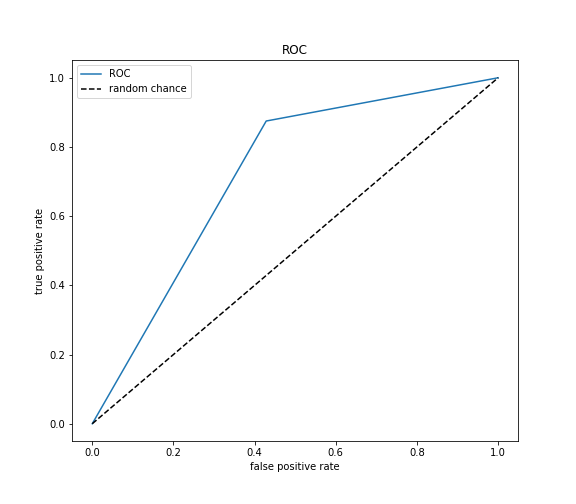####保存决策树桂枝图为pdf####
# 决策树规则生成dot对象
dot_data = export_graphviz(dt_model, max_depth=5, feature_names=dfs.columns[:-1], filled=True, rounded=True)

# 通过pydotplus将决策树规则解析为图形
graph = pydotplus.graph_from_dot_data(dot_data)

# 将决策树规则保存为PDF文件
graph.write_pdf('tree.pdf')
# 保存为jpg图片
graph.write_jpg('xx.jpg')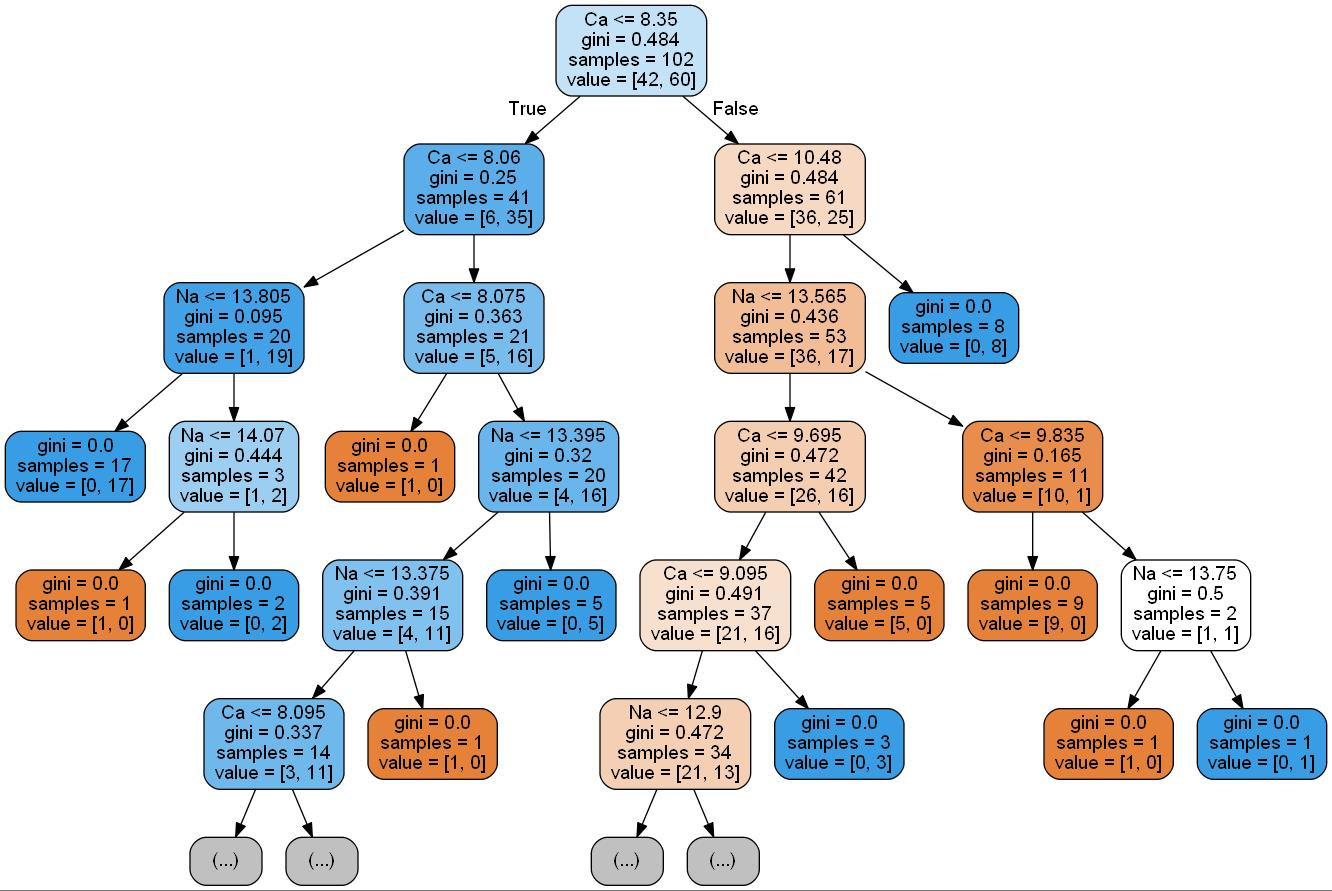## 决策树节点信息：

• rfm_score是分裂阈值，
• gini 是在当前规则下的基尼指数，
• nsamples是当前节点下的总样本量，
• nvalue为正例和负例的样本数量

## 混淆矩阵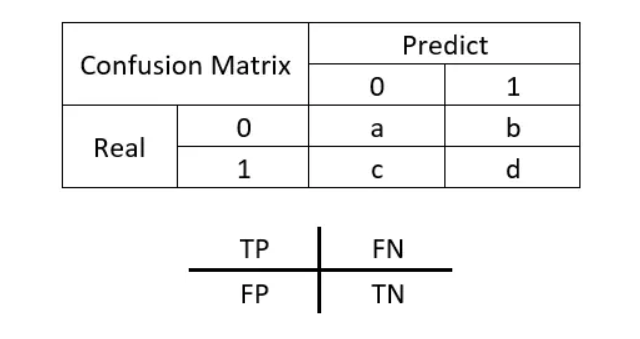• 真正（True Positive, TP）：本来是正例，分类成正例。
• 假正（True Negative, TN）：本来是负例，分类成负例。

• 假负（False Positive, FP）：本来是负例，分类成正例。
• 真负（False Negative, FN）：本来是正例，分类成负例。

## 评估指标解释：

• auc_s:AUC（Area Under Curve）, ROC曲线下的面积。ROC曲线一般位于y=x上方，因此AUC的取值范围一般在0.5和1之间。AUC越大，分类效果越好。
• accuracy_s：准确率（Accuracy），分类模型的预测结果中将正例预测为正例、将负例预测为负例的比例，公式为：A = (TP + TN)/(TP + FN + FP + TN)，取值范围[0,1]，值越大说明分类结果越准确。
• precision_s：精确度（Precision），分类模型的预测结果中将正例预测为正例的比例，公式为：P = TP/(TP+FP)，取值范围[0,1]，值越大说明分类结果越准确。
• recall_s：召回率（Recall），分类模型的预测结果被正确预测为正例占总的正例的比例，公式为：R = TP/(TP+FN)，取值范围[0,1]，值越大说明分类结果越准确。
• f1_s:F1得分（F-score），准确度和召回率的调和均值，公式为：F1 = 2 ＊ (P ＊R) / (P + R)，取值范围[0,1]，值越大说明分类结果越准确。
04-224万+12-204303
12-07266
11-10794
08-083241
09-211万+
05-234万+
02-068万+
12-13109
12-254895
01-07409
03-1844
08-242万+
10-041178
06-281万+
04-223524
10-12391
08-114905
04-19351
©️2020 CSDN 皮肤主题: 酷酷鲨 设计师:CSDN官方博客